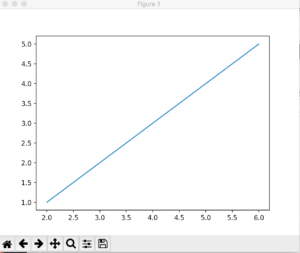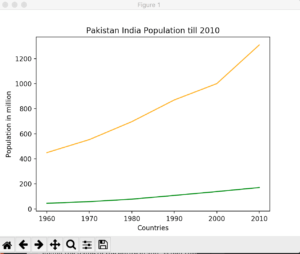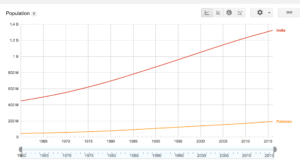I recently covered data gathering via scraping. Now I am going to cover how the data can be visualized. The best way to do is to plot graphs. Graphs makes it easier to see the relation between a data variable with other. There are various kinds of graphs available: Line, Bar, Chart, Histogram etc.

Since we are dealing in Python, it provides a very good library for plotting cool graphs. It’s called Matplotlib.

From the Official Site:

Matplotlib is a Python 2D plotting library which produces publication quality figures in a variety of hardcopy formats and interactive environments across platforms.

## Installation

The best way to install it by using `pip` command:

`pip install matplotlib`

`pip` will take care of dependences itself so you don’t need to get worried about it.

## Line Graph

So what is line graph? According to Wikipedia

A line chart or line graph is a type of chart which displays information as a series of data points called ‘markers’ connected by straight line segments.

Line graphs are usually used to find relationship between two data sets on different axis; for instance X, Y.

OK enough talk and now make our first graph program.

Create a new file, I call it `line.py` and import `matplotlib` library in it.

`import matplotlib.pyplot as plt`

The alias `plt` has been set for simplification purpose.

Next, we need our data points. Let’s take simple one:

```x = [2, 4, 6]
y = [1, 3, 5]
plt.plot(x, y)
plt.show()```

`plot()` takes various variables, for now I am passing `x` and `y` only for plotting purpose. The `show` method displays it in a window like below:As you can see, even numbers are are X-axis where odd on Y-axis.

You can also see a few buttons to manipulate the view of the chart, like Zoom button, moving forward and backward.

OK, it was very simple and to be honest a very useless graph. Let’s use some real dataset and plot the graph.

For sake of example  I am using population dataset of India and Pakistan. I am using Google Public Data as a data source.

```year = [1960, 1970, 1980, 1990, 2000, 2010]
pop_pakistan = [44.91, 58.09, 78.07, 107.7, 138.5, 170.6]
pop_india = [449.48, 553.57, 696.783, 870.133, 1000.
4, 1309.1]

plt.plot(year, pop_pakistan, color='g')
plt.plot(year, pop_india, color='orange')
plt.xlabel('Countries')
plt.ylabel('Population in million')
plt.title('Pakistan India Population till 2010')
plt.show()```

From the above source I got the data of both X and Y axis. `year` will be on x-axis where population of both countries. Since I need two different lines so `plot` was called twice. The `color` attribute used to assign the color of the line.

`xlabel` and `ylabel` are being used to give some friendly name to the axises and finally `.title()` for giving the name of the entire graph. When runs it will appear like given below:Looks cool, right? Pretty much similar to what you see on Google Public data interface, a bit of variance is because I rounded off the population for the sake of simplicity.That’s it for now. In coming post I will be discussing some other graph. Just hang in there.

## If you like this post then you should subscribe to my blog for future updates.

* indicates required